# Test Prep Plan - Take a practice test

Take this practice test to check your existing knowledge of the course material. We'll review your answers and create a Test Prep Plan for you based on your results.
How Test Prep Plans work
1
2Based on your results, we'll create a customized Test Prep Plan just for you!
3Study smarter
Study more effectively: skip concepts you already know and focus on what you still need to learn.

# 3rd Grade Math: Triangles Chapter Exam

Exam Instructions:

Choose your answers to the questions and click 'Next' to see the next set of questions. You can skip questions if you would like and come back to them later with the yellow "Go To First Skipped Question" button. When you have completed the practice exam, a green submit button will appear. Click it to see your results. Good luck!

### Page 1

#### Question 1 1. Identify x and y in the pictured triangle.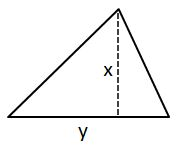#### Question 2 2. In the pictured triangle, what is side B, relative to angle x?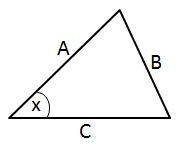#### Question 3 3. What type of triangle is pictured?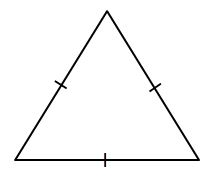### Page 2

#### Question 6 6. In the figure below, Angle 13 = 10y and Angle 11 = 3y + 11. Determine the measure of Angle 16.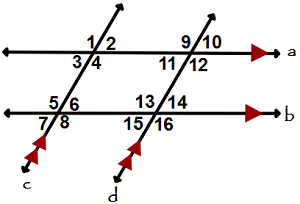#### Question 7 7. Is triangle ABC similar to triangle DEF?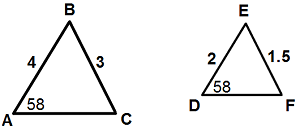#### Question 8 8. What type of triangle is pictured?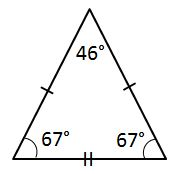#### Question 9 9. In the pictured triangle, what is side A, relative to angle x?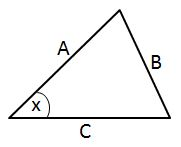#### Question 10 10. What type of triangle is pictured?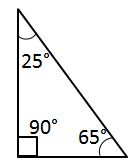### Page 3

#### Question 12 12. What kind of triangle is this?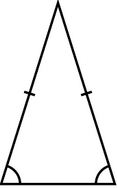#### Question 13 13. What kind of triangle is this?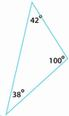#### Question 15 15. If angle a is 66 degrees and angle b is 54 degrees, what is the measure of angle d?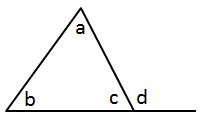### Page 4

#### Question 16 16. In the figure below, Angle 7 = 15x and Angle 10 = 9x + 30. Determine the measure of Angle 10.#### Question 17 17. What type of triangle is pictured?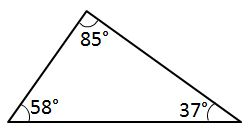#### Question 18 18. Given the triangle PQE, calculate the measure of Angle Q.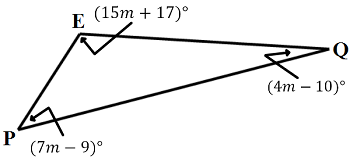#### Question 19 19. If angle a is 80 degrees, what is the measure of angle d?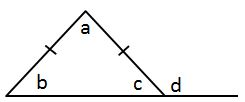#### Question 20 20. Josh claims that these triangles are similar by SSS. Do you agree or disagree with Josh?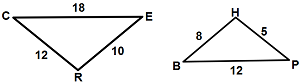### Page 5

#### Question 21 21. Conclude whether triangle BEN is similar to triangle MAT.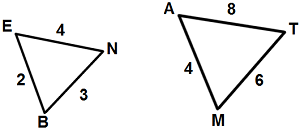#### Question 22 22. What type of triangle is pictured?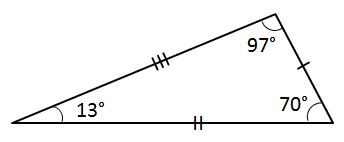#### Question 23 23. If angle a is 60 degrees, what is the measure of angle b?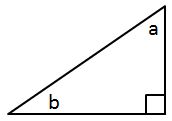#### Question 24 24. If angle a is 68 degrees and angle b is 37 degrees, what is the measure of angle c?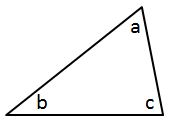### Page 6

#### Question 26 26. If angle a is 30 degrees and angle d is 70 degrees, what is the measure of angle b?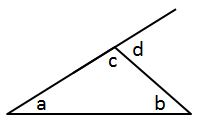#### Question 27 27. Determine if triangle VEZ is similar to triangle MAG.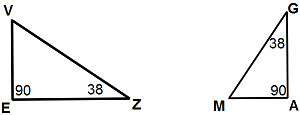#### Question 28 28. Given the triangle ABC, solve for the value of y.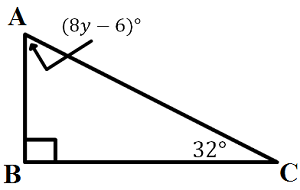#### Question 29 29. Mary claims that the triangles below are similar by AA. Do you agree or disagree with Mary?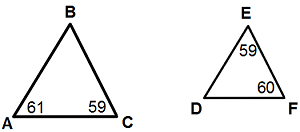#### Question 30 30. In triangle GBN, Angle G is seven more than three times a number, Angle B is five more than four times the number, and Angle N is twelve less than five times the number. Solve for the measure of Angle G.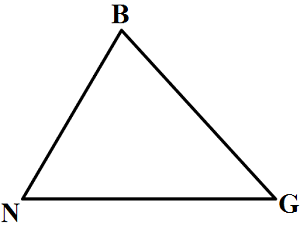#### 3rd Grade Math: Triangles Chapter Exam Instructions

Choose your answers to the questions and click 'Next' to see the next set of questions. You can skip questions if you would like and come back to them later with the yellow "Go To First Skipped Question" button. When you have completed the practice exam, a green submit button will appear. Click it to see your results. Good luck!

Support